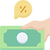top of page### Ratios

#### Leverage Ratios

###### Content ContributorsPriya KaurChristian Bien

# Learning Objectives###### ​###### What are Leverage / Gearing Ratios?Leverage / gearing ratios measure how much debt is within a business relative to its equity.

The Debt to Equity Ratio is the only ratio under the Leverage / Gearing category under the WACE syllabus.###### Debt to Equity RatioThe debt to equity ratio measures the amount of liabilities relative to its equity position. For example, a debt to equity ratio of 2 indicates that for every \$1 of equity, there is \$2 of liabilities.

Interpretations:

Ratio Below 1 - The business is classified as lowly geared as the equity position exceeds total liabilities.

Ratio Above 1 - The business is classified as highly geared as the total liability position exceeds equity.

Handy Tip: Comment with Times Interest Earned Comment on the debt to equity ratio with the Times Interest Earned Ratio. The Debt to equity ratio shows the level of gearing, while the Times Interest Earned ratio is an indicator of how effective that gearing is. For example, if a company had a debt to equity ratio of 3, but had a times interest earned ratio of 15 (above industry average), then it can be concluded that the highly geared position does not pose a risk as the times' interest earned ratio indicates the company is able to comfortably meet its debt arrangements.###### ​###### ​###### ​###### ​###### ​###### ​bottom of page